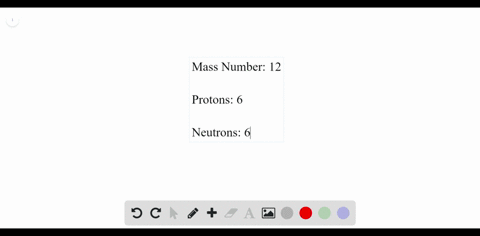### Carbon A carbon atom has a mass number of 12 and …

00:19University of Miami
Problem 66

How many protons and electrons are contained in an
atom of element 44 ?

Element 44 contains 44 protons as 44 electrons.

## Discussion

You must be signed in to discuss.

## Video Transcript

So now we'll work on problem 66 from Chapter four. This problem were asked about how many protons and electrons would be in an Adam of Element 44. So Element 44 means that the atomic number is equal to 40 44. We know that atomic number is equal to the number of protons, and in a neutral atoms that's also equal to the number of electrons. So the answer before protons and electrons must be the atomic number, which we're told is 44.